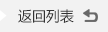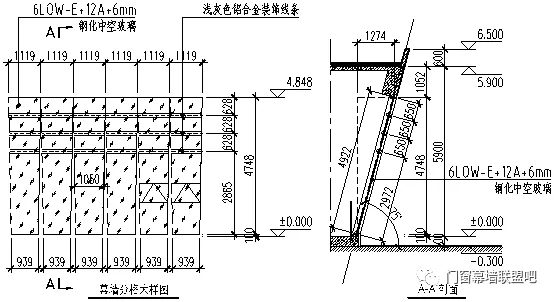﻿ 浅谈斜玻璃幕墙的计算一

#13431191194 0755-2738-6668

# 浅谈斜玻璃幕墙的计算一来源：中东幕墙 查看手机网址

1. 计算简图1. 计算单元选取

2. 风荷载计算

3. 面板玻璃计算

1. 中空玻璃强度校核：

σ: 外侧玻璃所受应力:

σ=1.4×σWK+0.5×1.3×σEK×（1/ sin α）

σ: 内侧玻璃所受应力:

σ=1.4×σWK+0.5×1.3×σEK×（1/ sin α）

4. 固定片（压板）计算

5. 硅酮结构密封胶计算

1. 硅酮结构密封胶宽度的计算:

Cs1: 结构胶在风载荷作用下的粘结宽度 (mm)

Cs1=(W+0.5×qE)×a/(2000×f1×（1/ sin α）

Cs2: 结构胶在自重效应下的粘结宽度 (mm)

Cs2=1.35×25.6×(Bt_l+Bt_w)×(a×b)/(2000×(a+b)×f2) ×（1/ sin α）

2. 胶缝厚度在水平风荷载作用下的校核:

ts: 结构胶在风荷载作用下的粘结厚度

ts=θ×hg×ψ/(δ2×(2+δ2))0.5×（1/ sin α）

6. 幕墙立柱计算

1. 幕墙立柱强度的校核:

A. 内套承载力计算:

B. 外套承载力计算:

校核依据: N×10/An+M·Ea·Ya1/(Ea·Ia+Es·Is)/1.05×（1/ sin α）

2. 幕墙立柱刚度的校核:

df: 立柱最大挠度

df=5×qWk×Hsjcg4×1000)/(384×(Ea×Ia+Es×Is)) ×（1/ sin α）

3. 立柱与主结构连接:

N: 连接处总合力(N):

N=(N12+N22)0.5×（1/ sin α）

7. 幕墙预埋件截面面积计算

As1=V/(αr×αv×fy)+N/(0.8×αb×fy)+M/(1.3×αr×αb×fy×Z) ×（1/ sin α）

As2=N/(0.8×αb×fy)+M/(0.4×αr×αb×fy×Z) ×（1/ sin α）

8. 幕墙预埋件焊缝计算

((σff)2f2)0.5×（1/ sin α）

9. 幕墙横梁计算

1. 横梁剪应力的校核:

τx=Vy×Sx/(Ix×tx×（1/ sin α）

τy=Vx×Sy/(Iy×ty×（1/ sin α）

2. 横梁刚度的校核:

dfw=qwk×Wfg4×1000/(0.7×Ix×120) ×（1/ sin α）

dfG=5×GK×Wfg4×1000/(384×0.7×Iy×（1/ sin α）

10. 横梁与立柱连接件计算

1. 横梁与立柱间连结

(1)横向节点(横梁与角码)

N1: 连接部位受总剪力:

N1=(Qw+0.5×QE)×1000×（1/ sin α）

(2)竖向节点(角码与立柱)

N: 连接处组合荷载:

N=(N12+N22)0.5×（1/ sin α）

#### “推荐阅读”

【本文标签】：玻璃幕墙
【责任编辑】：中东幕墙版权所有：http://www.zdmq88.com转载请注明出处